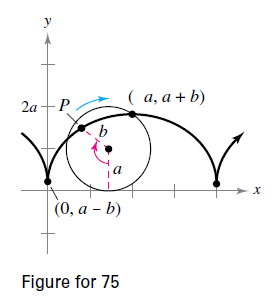Chapter 10.2, Problem 73E

Chapter
Section
Textbook Problem

# Curtate Cycloid A wheel of radius a rolls along a line without slipping. The curve traced by a point P that it b units front the center ( b < a ) is called a curtate cycloid (see figure). Use the angle θ to find a set of parametric equations for this curve.To determine
The set of parametric equation for the Curate Cycloid.

Explanation

Given: A wheel of radius a roll without slipping, the curve traced by the point

P is b units from the centre.

Explanation:

As pointed out in the figure, the point P is assumed to be at (x, y).

Now, x co-ordinate is given by OR = OW - RW=aθsin(SPO).

= aθbsin(180;

### Still sussing out bartleby?

Check out a sample textbook solution.

See a sample solution

#### The Solution to Your Study Problems

Bartleby provides explanations to thousands of textbook problems written by our experts, many with advanced degrees!

Get Started

#### Differentiate. y=x2tanx

Calculus: Early Transcendentals

#### Solve the equations in Exercises 126. x4x+1xx1=0

Finite Mathematics and Applied Calculus (MindTap Course List)

#### Finding a Limit In Exercises 47-62, find the limit. limx4x4x216

Calculus: Early Transcendental Functions (MindTap Course List)